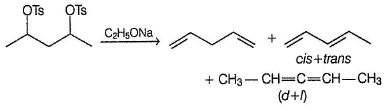Courses

# Test: Reaction Of Alcohols- I

## 19 Questions MCQ Test Chemistry for JEE | Test: Reaction Of Alcohols- I

Description
This mock test of Test: Reaction Of Alcohols- I for JEE helps you for every JEE entrance exam. This contains 19 Multiple Choice Questions for JEE Test: Reaction Of Alcohols- I (mcq) to study with solutions a complete question bank. The solved questions answers in this Test: Reaction Of Alcohols- I quiz give you a good mix of easy questions and tough questions. JEE students definitely take this Test: Reaction Of Alcohols- I exercise for a better result in the exam. You can find other Test: Reaction Of Alcohols- I extra questions, long questions & short questions for JEE on EduRev as well by searching above.
QUESTION: 1

### Only One Option Correct Type Direction (Q. Nos. 1-8) This section contains 8 multiple choice questions. Each question has four choices (a), (b), (c) and (d), out of which ONLY ONE is correct. Q.  The correct order of acidic strength of the following alcohols is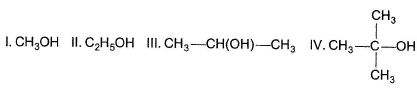Solution:

Electron donating (+ / -effect) alkyl groups decreases the acidic strength.

QUESTION: 2

Solution:
QUESTION: 3

### Which compound given below has the highest solubility in water ?

Solution:

Greater the number of hydroxy groups , greater its a bility to form H-bonds with water, hence greater solubility.

QUESTION: 4

What is the correct increasing order of acidity of the following?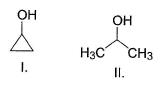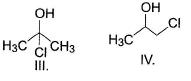Solution:

Both (I) and (II) are 2° alcohols, less acidicthan (I) and (IV) where there is electron withdrawing chloride group is present. Between (I) and (II), (I) is less acidic as ring is considered here equivalent to two ethyl groups attached to α-carbon. (Ill) is more acidic than (IV) because in (III) Cl is at α-carbon while it is at β-carbon in (IV).

QUESTION: 5

What is the order of solubility of the following in water?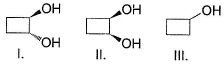Solution:

Dihydric alcohols are always more soluble in water than monohydric alcohol. Between (I) and (II), (I) is more soluble as it forms intermolecular H-bonds with water while (II) forms intramolecular H-bonds which decreases its ability to form intermolecular H-bonds with water.

QUESTION: 6

Which of the following can be used for the distinction of ethanol from phenol?

Solution:

Phenol forms salt with NaOH while a liphatic alcohols do not.

QUESTION: 7

Consider the following reaction,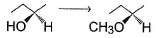The above reaction can best be brought about by

Solution:

In the below reaction , no bond with chiral carbon is disturbed, hence, configuration is retained in product.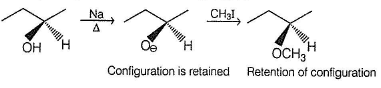QUESTION: 8

Consider the following reaction,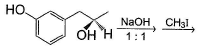The major product is

Solution:

Phenolic—OH is neutralised here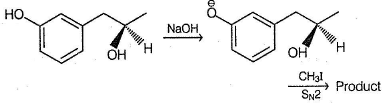*Multiple options can be correct
QUESTION: 9

One or More than One Options Correct Type

Direction (Q. Nos. 9-12) This section contains 4 multiple choice questions. Each question has four choices (a), (b), (c) and (d), out of which ONE or MORE THAN ONE are correct.

Consider the compound shown below,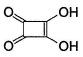Select the correct statement(s).

Solution:

Methanol is a liquid chemical with the formula CH3​OH. It is colourless, volatile, flammable and poisonous.

Methanol is made from the destructive distillation of wood and is chiefly synthesized from carbon monoxide and hydrogen.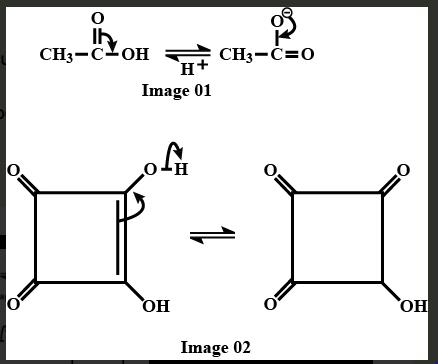It is more acidic compound bc2​ this molecule loss H+ ions very easily. as compared to compound [A]

It reacts very difficult to lucas reagent process via SN1 mechanism which depends on carbocation stability.

It is diacid base loss H+ easily.

*Multiple options can be correct
QUESTION: 10

Methanol and ethanol can be distinguished by

Solution: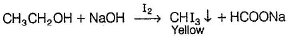No such reaction occur with CH3OH.
Acidic KMnO4 forms CO2 with methanol but CH3COOH with ethanol.

*Multiple options can be correct
QUESTION: 11

Consider the following substituted ethanol G—CH2CH2OH

Which group(s) when present as G gives greater equilibrium of gauche conformer than its anti counterpart ?

Solution:

All these groups increases the stability of gauche conformer due to stable intramolecular H-bonding.

*Multiple options can be correct
QUESTION: 12

Which of the statement given below concerning 3-methyl-2-butanol is/are correct ?

Solution: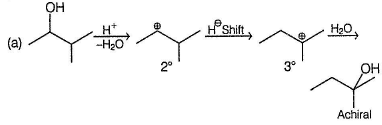(c) PCI5 brings about SN2 reaction, chloro alkane with inverted configuration would be produced.
(d) 3-methyl-2-butanol is a secondary alcohol, will be oxidised to ketone with acidic dichromate solution.

QUESTION: 13

Comprehension Type

Direction (Q. Nos. 13-15) This section contains a paragraph, describing theory, experiments, data, etc. Three questions related to the paragraph have been given. Each question has only one correct answer among the four given options (a), (b), (c) and (d).

Passage

An organic compound X (C10H12O3) is not soluble in water or NaHCO3. A solution of Br2 in CCI4 is decolourised by X forming C10H12O3Br2. X on controlled ozonolysis followed by the treatm ent with (CH3)2S gives Y (C8H8O3) and C2H4O2. Y can also be obtained by reaction between ortho methoxy phenol with CHCI3 in KOH solution followed by acid hydrolysis.

Q.

What is the correct structure of X?

Solution:

The key point is formation of Y from ortho methoxy phenol by Reimer-Tiemann reaction.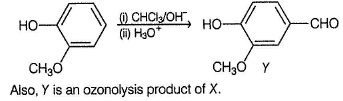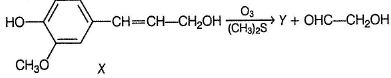QUESTION: 14

An organic compound X (C10H12O3) is not soluble in water or NaHCO3. A solution of Br2 in CCI4 is decolourised by X forming C10H12O3Br2. X on controlled ozonolysis followed by the treatm ent with (CH3)2S gives Y (C8H8O3) and C2H4O2. Y can also be obtained by reaction between ortho methoxy phenol with CHCI3 in KOH solution followed by acid hydrolysis.

Q.

If X is treated with cold HBr, the major product would be

Solution:

The key point is formation of Y from ortho methoxy phenol by Reimer-Tiemann reaction.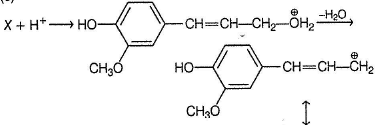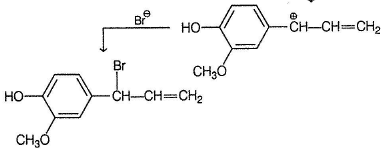QUESTION: 15

An organic compound X (C10H12O3) is not soluble in water or NaHCO3. A solution of Br2 in CCI4 is decolourised by X forming C10H12O3Br2. X on controlled ozonolysis followed by the treatm ent with (CH3)2S gives Y (C8H8O3) and C2H4O2. Y can also be obtained by reaction between ortho methoxy phenol with CHCI3 in KOH solution followed by acid hydrolysis.

Q.

What would be the major product if X is treated with cold concentrated H2SO4?

Solution:

The key point is formation of Y from ortho methoxy phenol by Reimer-Tiemann reaction.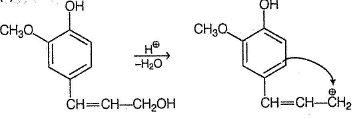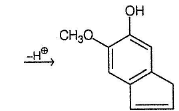*Answer can only contain numeric values
QUESTION: 16

One Integer Value Correct Type

Direction (Q. Nos. 16-19) This section contains 4 questions. When worked out w ill result in an integer from 0 to 9 (both inclusive).

Q.

How many reagents from the list given below, gives visible change when treated with 2-propanol?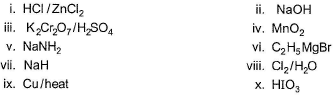Solution:

(i) IHCI/ZnCI2 (Lucas reagent) gives white turbidity.
(ii) NaOH does not give any visible change.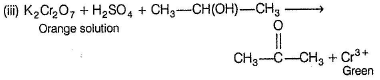(iv) MnO2 does not oxidises 2-propanol.
(v) NaNH2 gives effervescence of NH3,
(vi) C2H5 MgBrgives C2H6(g)
(vii) NaH gives H2(g)
(viii), (ix) and (x) all react with 2-propanol but no visible change would be observed.
Hence, only (i), (iii), (v), (vi) and (vii) gives visible change.

*Answer can only contain numeric values
QUESTION: 17

If 4-methyl-2-pentene is refluxed with dilute H2SO4, hydration reaction takes place. In principle, how many different alcohols are formed ?

Solution: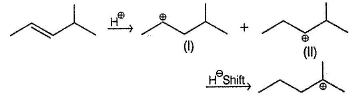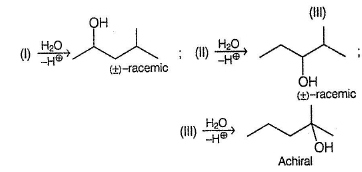*Answer can only contain numeric values
QUESTION: 18

Consider the following reaction, how many different organic products are formed at the end of the reaction?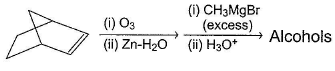Solution: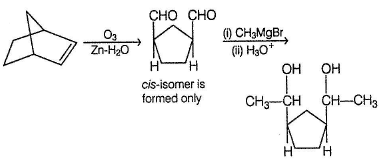Three stereomers of final product are formed, a meso and a pair of enantiomers.

*Answer can only contain numeric values
QUESTION: 19

If pentane-2, 4-diol is treated with excess of p-toluene sulphonyl chloride followed by C2H5ONa/C2H5OH, dienes are formed by E2 elimination reaction. In principle, how many different dienes are formed ?

Solution: